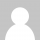这段只有1K的代码能做什么？

with(m=Math)C=cos,S=sin,P=pow,R=random;c.width=c.height=f=500;h=-250;function p(a,b,c){if(c>60)return[S(a*7)*(13+5/(.2+P(b*4,4)))-S(b)*50,b*f+50,625+C(a*7)*(13+5/(.2+P(b*4,4)))+b*400,a*1-b/2,a];A=a*2-1;B=b*2-1;if(A*A+B*B<1){if(c>37){n=(j=c&1)?6:4;o=.5/(a+.01)+C(b*125)*3-a*300;w=b*h;return[o*C(n)+w*S(n)+j*610-390,o*S(n)-w*C(n)+550-j*350,1180+C(B+A)*99-j*300,.4-a*.1+P(1-B*B,-h*6)*.15-a*b*.4+C(a+b)/5+P(C((o*(a+1)+(B>0?w:-w))/25),30)*.1*(1-B*B),o/1e3+.7-o*w*3e-6]}if(c>32){c=c*1.16-.15;o=a*45-20;w=b*b*h;z=o*S(c)+w*C(c)+620;return[o*C(c)-w*S(c),28+C(B*.5)*99-b*b*b*60-z/2-h,z,(b*b*.3+P((1-(A*A)),7)*.15+.3)*b,b*.7]}o=A*(2-b)*(80-c*2);w=99-C(A)*120-C(b)*(-h-c*4.9)+C(P(1-b,7))*50+c*2;z=o*S(c)+w*C(c)+700;return[o*C(c)-w*S(c),B*99-C(P(b, 7))*50-c/3-z/1.35+450,z,(1-b/1.2)*.9+a*.1, P((1-b),20)/4+.05]}}setInterval('for(i=0;i<1e4;i++)if(s=p(R(),R(),i%46/.74)){z=s;x=~~(s*f/z-h);y=~~(s*f/z-h);if(!m[q=y*f+x]|m[q]>z)m[q]=z,a.fillStyle="rgb("+~(s*h)+","+~(s*h)+","+~(s*s*-80)+")",a.fillRect(x,y,1,1)}',0)

8 Responses to 这段只有1K的代码能做什么？

1.leftboy says:

呵呵，之前看到过VB、VC版本的电脑全屏玫瑰花的程序…现在又来了这个版本，不错…

2.HH says:

技术宅准备的情人节礼物么。。。

3.Aaron says:

据说帝国时代这个游戏就是用html5写的。

4.路過 says:

但是它好像不会自娱停止，
只要在这一页面，cpu就跑不停

5.bzhao says:

perfect

6.dayu says:

很强大，要回那么多的知识。。。

7.haitao says:

js代码已经会很容易混乱的了，还玩“比谁混乱”？

8.SmilePeter says:

o(∩_∩)o 哈哈~~很赞啊，师兄~~~